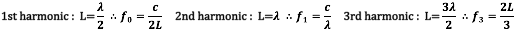# Waves

Properties, polarisation, stationary waves

Waves

Types of Waves

·      Longitudinal waves are caused by a vibration that is parallel to the direction of the wave e.g. sound

·      Transverse waves are caused by a vibration that is perpendicular to the direction of the wave e.g. electromagnetic waves, waves on a string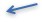Properties of Waves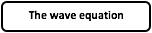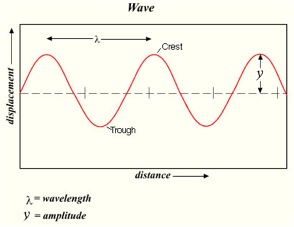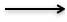c= wave speed (or velocity)

f = frequency (Hz) – how many waves pass a point per second

_ = wavelength (m)

T = time period – time for one complete wave to pass a point (s)

Phase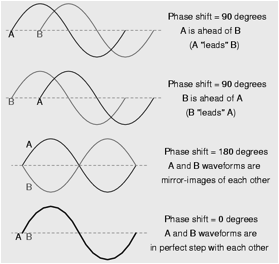·      Sometimes we also use the term ‘path difference’ to describe the difference between two waves

for example – the red wave here has travelled a distance of 1 wavelength or _ further than the orange wave – but they are still in phase (phase difference of 0 or 3600

·      A path difference of _/2 would make 2 identical waves 180 out of phasePolarisation

·      Only transverse waves have polarisation

·      It is a measure of the angle of vibration as viewed along the axis of the wave

·      Unpolarised waves have all angles present

·      A polaroid is a material that can polarise light in one angle

Wave FrontsWaves produced by a ripple tank are called wave fronts

Each line represents the crest of a wave

The distance between two wave fronts in the wavelength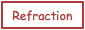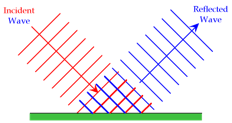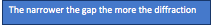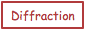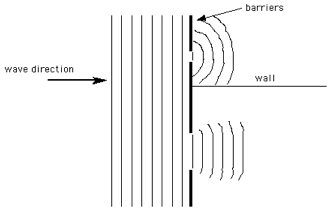Superposition

·      The principle of superposition is that when two waves meet, the total displacement at a point is equal to the sum of the individual displacements at that pointStationary Waves

·      A wave that moves through a medium and carries energy from one place to another is called a progressive wave

·      A stationary wave (sometimes referred to as a ‘standing wave’)

-   Does not transfer energy (it stores it)

-   Is formed when two progressive waves pass through each other in opposite directions

-   When the waves have the same frequency/wavelength and similar amplitude

-   Can be formed in many different places e.g. on a vibrating string, by sound in a tube, by microwaves reflecting from metal plates

-   Only occur when the progressive waves have certain frequencies

Imagine two progressive waves of equal wavelength/frequency and the same amplitude meeting from opposite directions.: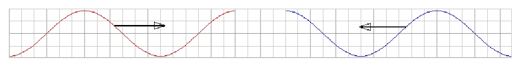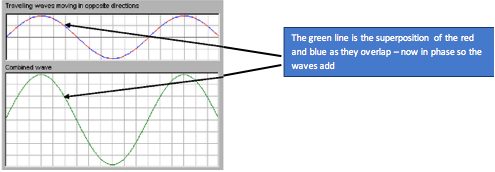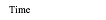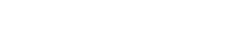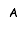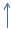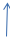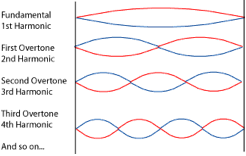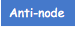Using the wave equation and the wavelength of the wave compared to the length of the gap gives formula for the frequency of waves which will produce stationary waves: